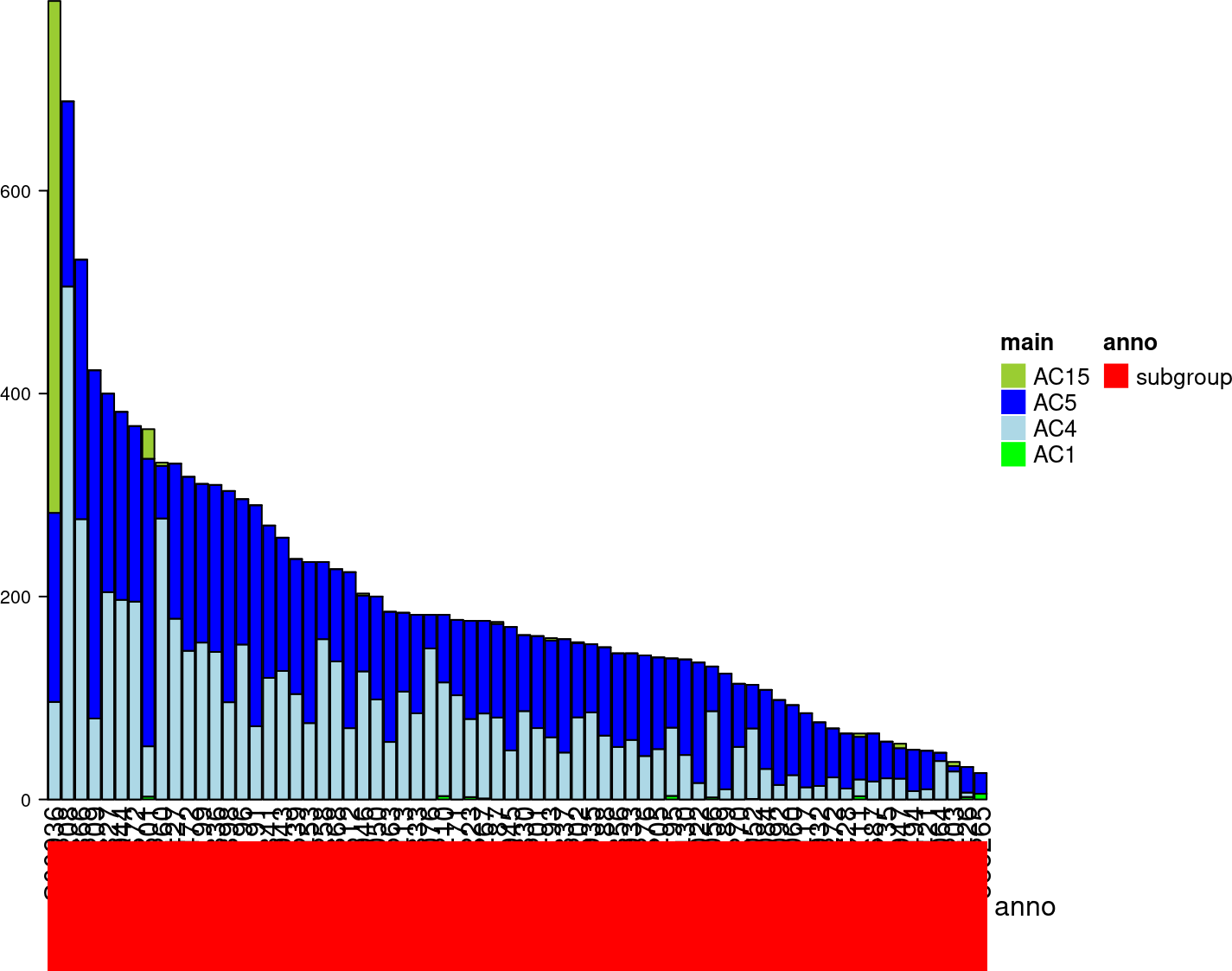# Contents

library(BiocStyle)

# 1 Introduction

The usage of YAPSA for Whole Genome Sequencing (WGS) data has been described in detail in the preceding vignettes, with an introduction and an overview of the general framework in 1. Usage of YAPSA. YAPSA can also be applied to Whole Exome Sequencing (WES) data, however, a few caveats apply and a few steps have to be followed. These are described in this vignette.

The most important difference between WGS and WES analyses is the frequency of occurrence of the different k-mers. According to the concept detailed in (Alexandrov et al. 2013) and (Alexandrov et al. 2020), SNV mutational signatures use the triplet (or 3-mer) context of an SNV for categorization of the mutations, leading to 96 different categories or features. These 96 different feature don’t occur with the same frequency in the human genome. They don’t occur with the same frequency in the exome either, but more importantly, the relative occurrence differs between WGS and WES. More precisely, let $$n_{X}^{WGS}$$ denote the occurrence of feature $$X$$ in the whole genome and $$n_{X}^{WES}$$ denote the occurrence of $$X$$ in an exome target capture. We then furthermore denote

1. $q_{X}^{WGS,WES} = \frac{n_{X}^{WGS}}{n_{X}^{WES}}$

to be the ratio of these two counts. Even though on average across all features, these ratios may vary around a value of $$50$$, because the genome is roughly $$50$$ times bigger than the exome, the ratios need not be identical for all features, i.e.,

1. $\exists X,Y\in\mathbb{F}: q_{X}^{WGS,WES} \neq q_{Y}^{WGS,WES}$

where $$\mathbb{F}$$ denotes the feature space. It is thus crutial to compute $$q_{X}^{WGS,WES}$$ for all features $$X\in\mathbb{F}$$ and to correct for these differences. These corrections can be applied either to the signatures, converting them to “exome signatures”, or the inverse corrections can be applied to the mutational catalogs. In YAPSA, we opt for the second alternative, as this keeps the function calls simple, analogous and very similar for analyses of both WES and WGS data.

Different target capture kits are available in order to perform WES. As these cover different genomic regions, for a given target capture kit $$A$$, different correction factors $$q_{X}^{WGS,WES_A}$$ for all features have to be computed. As detailed below, YAPSA provides correction factors for 8 different target capture kits and also one correction factor directly derived from the gene model GENCODE 19 applied to the human reference genome hs37d5.

First of all, analogously to all other vignettes, we load the signature data from the package:

data(sigs)
data(cutoffs)
current_sig_df <- AlexInitialArtif_sig_df
library(BSgenome.Hsapiens.UCSC.hg19)

For the purpose of analyzing exomes, a mutational catalog of small cell lung cancer is stored in YAPSA. The data had originally been generated by (Rudin et al. 2012) and was used in the cross entity analysis in (Alexandrov et al. 2013). The data can be accessed as follows:

data("smallCellLungCancerMutCat_NatureGenetics2012")

This creates a dataframe with name exome_mutCatRaw_df and 96 rows. It was originally generated by executing the R code below (not evaluated in this vignette):

smallCellLungCancer_NatureGenetics2012_ftp_path <- paste0(
"ftp://ftp.sanger.ac.uk/pub/cancer/AlexandrovEtAl/",
"somatic_mutation_data/Lung Small Cell/",
"Lung Small Cell_clean_somatic_mutations_for_signature_analysis.txt")
exome_vcf_like_df <-
names(exome_vcf_like_df) <- c("PID","TYPE","CHROM","START",
"STOP","REF","ALT","FLAG")
exome_vcf_like_df <- subset(exome_vcf_like_df, TYPE == "subs",
select = c(CHROM, START, REF, ALT, PID))
names(exome_vcf_like_df) <- "POS"
exome_vcf_like_df <- translate_to_hg19(exome_vcf_like_df,"CHROM")
word_length <- 3
exome_mutCatRaw_list <-
create_mutation_catalogue_from_df(
exome_vcf_like_df,
this_seqnames.field = "CHROM", this_start.field = "POS",
this_end.field = "POS", this_PID.field = "PID",
this_subgroup.field = "SUBGROUP",
this_refGenome = BSgenome.Hsapiens.UCSC.hg19,
this_wordLength = 3)
exome_mutCatRaw_df <- as.data.frame(exome_mutCatRaw_list$matrix) # 3 Correcting for the target capture We now have an example mutational catalog at hand for WES data. In order to perform a correction for the different triplet content between WGS and WES, YAPSA provides correction factors, which can be brought to the R workspace as follows: data(targetCapture_cor_factors) As outlined in the introduction, different target capture kits require different correction factors. The sets of available correction factors can be accessed by the names of the loaded object: names(targetCapture_cor_factors) ##  "Agilent4withUTRs" "Agilent4withoutUTRs" ##  "Agilent5withUTRs" "Agilent5withoutUTRs" ##  "SomSig" "hs37d5" ##  "IlluminaNexteraExome" "Agilent6withoutUTRs" ##  "Agilent6withUTRs" "Agilent7withoutUTRs" ##  "AgilentSureSelectAllExon" We now have everything at hand which is needed to correct for the triplet content. As described above, the data was accessed via links provided in (Alexandrov et al. 2013), however, the data had originally been generated for another publication: (Rudin et al. 2012). As described there, the target capture kit Agilent SureSelect all exon was used, and we will use the correction factors computed for this very kit. The function to be used in YAPSA for such a correction is called normalizeMotifs_otherRownames(): targetCapture <- "AgilentSureSelectAllExon" cor_list <- targetCapture_cor_factors[[targetCapture]] corrected_catalog_df <- normalizeMotifs_otherRownames(exome_mutCatRaw_df, cor_list$rel_cor)

Of note, the corrected mutational catalog need not contain exclusively integer numbers any more, it may contain floating point numbers due to the values of $$q_{X}^{WGS,WES}$$.

# 4 Performing the analysis of mutational signatures

After having performed the corrected with the factors $$q_{X}^{WGS,WES}$$, the analysis of mutational signatures is completely analogous to the steps already described multiple times in the other vignettes. However, the choice of optimal signature-specific cutoffs is slightly different:

data(cutoffs)
current_cutoff_vector <- cutoffCosmicValid_rel_df[6,]
exome_listsList <-
LCD_complex_cutoff_combined(
in_mutation_catalogue_df = corrected_catalog_df,
in_cutoff_vector = current_cutoff_vector,
in_signatures_df = AlexCosmicValid_sig_df,
in_sig_ind_df = AlexCosmicValid_sigInd_df)

As we don’t have any information about subgroups in this example, we omit this and proceed directly with plotting the results:

exposures_barplot(
in_exposures_df = exome_listsList$cohort$exposures,
in_signatures_ind_df = exome_listsList$cohort$out_sig_ind_df)Figure 1: Exposures barplot

Of note, this cohort is strongly affected by signature AC4 (associated with the main carcinogens in tobacco smoke).